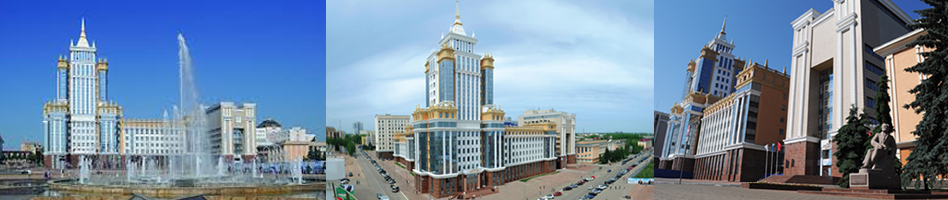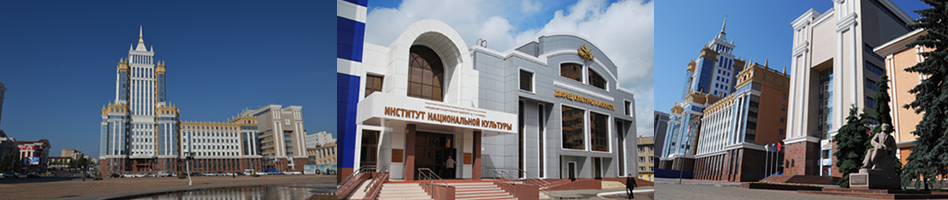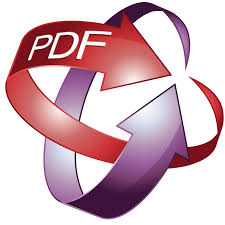To download article.

UDC 531.1:519.854

DOI: 10.15507/0236-2910.027.201702.224-238

Quantum superposition of the state discrete spectrum of mathematical correlation molecule for small samples of biometric data

President of the Penza State University (40 Krasnaya St., Penza 440000 Russia), Dr.Sci. (Engineering), professor, This email address is being protected from spambots. You need JavaScript enabled to view it.

Aleksandr I. Ivanov
Head of the Laboratory of Biometric and Neural Network Technologies, Penza Electrotechnical Research Institute (9 Sovetskaya St., Penza 440026, Russia), Dr.Sci. (Engineering), docent, ORCID: http://orcid.org/0000-0002-3854-2660, This email address is being protected from spambots. You need JavaScript enabled to view it.

Andrey V. Serikov
Head of Department of Joint-Stock Company Rubin (2 Baydukova St., Penza 440000, Russia), ORCID: http://orcid.org/0000-0002-6870-3349, This email address is being protected from spambots. You need JavaScript enabled to view it.

Yuliya I. Serikova
Graduate Student of the Penza State University (40 Krasnaya St., Penza 440000 Russia), ORCID: http://orcid.org/0000-0002-4959-321X , julia-ska@yandex.r

Introduction: The study promotes to decrease a number of errors of calculating the correlation coefficient in small test samples.
Materials and Methods: We used simulation tool for the distribution functions of the density values of the correlation coefficient in small samples. A method for quantization of the data, allows obtaining a discrete spectrum states of one of the varieties of correlation functional. This allows us to consider the proposed structure as a mathematical correlation molecule, described by some analogue continuous-quantum Schrödinger equation.
Results: The chi-squared Pearson’s molecule on small samples allows enhancing power of classical chi-squared test to 20 times. A mathematical correlation molecule described in the article has similar properties. It allows in the future reducing calculation errors of the classical correlation coefficients in small samples.
Discussion and Conclusions: The authors suggest that there are infinitely many mathematical molecules are similar in their properties to the actual physical molecules. Schrödinger equations are not unique, their analogues can be constructed for each mathematical molecule. You can expect a mathematical synthesis of molecules for a large number of known statistical tests and statistical moments. All this should make it possible to reduce calculation errors due to quantum effects that occur in small test samples.

Keywords: correlation coefficient, quantum superposition, molecule, discrete spectrum states, statistical analysis of small samples

For citation: Volchikhin V. I., Ivanov A. I., Serikov A. V., Serikova Yu. I. Quantum superposition of the state discrete spectrum of mathematical correlation molecule for small samples of biometric data. Vestnik Mordovskogo universiteta = Mordovia University Bulletin. 2017; 2(27):224-238. DOI: 10.15507/0236-2910.027.201702.224-238

Contribution of the co-authors: V. Volchikhin: scientific supervision, modernization of the quantum-mechanical paradigm; A. Ivanov: formalization of requirements for multidimensional biometric data, formalization of requirements for the volume of test samples; A. Serikov: synthesis of the mechanism of quantization of the two-dimensional continuum of internal states of the correlation molecule; Yu. Serikova: software implementation, calculation of position and amplitude of spectral lines.